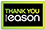# Schaum's outline of differential equations

PAPERBACK
Study smarter and stay on top of your differential equations course with the bestselling Schaum's Outline-now with the NEW Schaum's app and website! Schaum's Outline of Differential Equations, Fifth Edition is the go-to study guide for all students of science who need to learn or refresh their knowledge of differential equations. With an outline format that facilitates quick and easy review and mirrors the course in scope and sequence, this book helps you understand basic concepts and get the extra practice you need to excel in the course. It supports the all major differential equations textbooks and is useful for study in Calculus (I, II, and III), Mathematical Modeling, Introductory Differential Equations and Differential Equations. Chapters include an Introduction to Modeling and Qualitative Methods, Classifications of First-Order Differential Equations, Linear Differential Equations, Variation of Parameters, Initial-Value Problems for Linear Differential Equations, Graphical and Numerical Methods for Solving First-Order Differential Equations, Solutions of Linear Differential Equations with Constant Coefficients by Laplace Transforms, and more. Features: NEW to this edition: the new Schaum's app and website!NEW CHAPTERS include Autonomous Differential Equations and Qualitative Methods; Eigenvalues and Eigenvectors; three chapters dealing with Solutions of Systems of Autonomous Equations via Eigenvalues and Eigenvectors (real and distinct, real and equal, and complex conjugate Eigenvalues)20 problem-solving videos online563 solved problemsOutline format provides a quick and easy review of differential equationsClear, concise explanations of differential equations conceptsHundreds of examples with explanations of key conceptsSupports all major textbooks for differential equations coursesAppropriate for the following courses: Calculus (I, II, and III), Mathematical Modeling, Introductory Differential Equations, and Differential Equations
€25.1975 Reward Points
In stock online
Delivery in 2-3 working days
Free Delivery on this item

Any purchases for more than €10 are eligible for free delivery anywhere in the UK or Ireland!

Study smarter and stay on top of your differential equations course with the bestselling Schaum's Outline-now with the NEW Schaum's app and website! Schaum's Outline of Differential Equations, Fifth Edition is the go-to study guide for all students of science who need to learn or refresh their knowledge of differential equations. With an outline format that facilitates quick and easy review and mirrors the course in scope and sequence, this book helps you understand basic concepts and get the extra practice you need to excel in the course. It supports the all major differential equations textbooks and is useful for study in Calculus (I, II, and III), Mathematical Modeling, Introductory Differential Equations and Differential Equations. Chapters include an Introduction to Modeling and Qualitative Methods, Classifications of First-Order Differential Equations, Linear Differential Equations, Variation of Parameters, Initial-Value Problems for Linear Differential Equations, Graphical and Numerical Methods for Solving First-Order Differential Equations, Solutions of Linear Differential Equations with Constant Coefficients by Laplace Transforms, and more. Features: NEW to this edition: the new Schaum's app and website!NEW CHAPTERS include Autonomous Differential Equations and Qualitative Methods; Eigenvalues and Eigenvectors; three chapters dealing with Solutions of Systems of Autonomous Equations via Eigenvalues and Eigenvectors (real and distinct, real and equal, and complex conjugate Eigenvalues)20 problem-solving videos online563 solved problemsOutline format provides a quick and easy review of differential equationsClear, concise explanations of differential equations conceptsHundreds of examples with explanations of key conceptsSupports all major textbooks for differential equations coursesAppropriate for the following courses: Calculus (I, II, and III), Mathematical Modeling, Introductory Differential Equations, and Differential Equations
Quantity:
In stock online
Delivery in 2-3 working days
Free Delivery on this item75 Reward Points

Any purchases for more than €10 are eligible for free delivery anywhere in the UK or Ireland!

€25.19
In stock online
Delivery in 2-3 working days
Free Delivery on this item
Quantity:75 Reward Points

Any purchases for more than €10 are eligible for free delivery anywhere in the UK or Ireland!